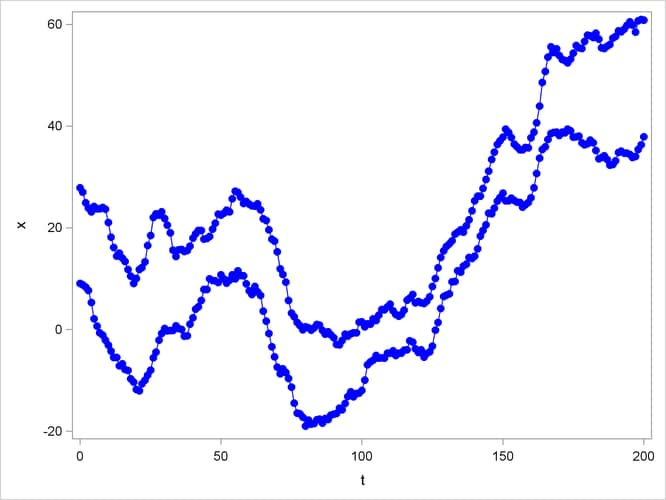The STATESPACE Procedure

Getting Started: STATESPACE Procedure

Subsections:

The following introductory example uses simulated data for two variables X and Y. The following statements generate the X and Y series.

```data in;
x=10;  y=40;
x1=0; y1=0;
a1=0; b1=0;
iseed=123;
do t=-100 to 200;
a=rannor(iseed);
b=rannor(iseed);
dx = 0.5*x1 + 0.3*y1 + a - 0.2*a1 - 0.1*b1;
dy = 0.3*x1 + 0.5*y1 + b;
x = x + dx + .25;
y = y + dy + .25;
if t >= 0 then output;
x1 = dx; y1 = dy;
a1 = a; b1 = b;
end;
keep t x y;
run;
```

The simulated series X and Y are shown in Figure 28.1.

Figure 28.1: Example Series